# Gnuplotting

Create scientific plots using gnuplot

April 13th, 2015 | 3 Comments

Instead of using a legend it is often a good idea to label your data directly in the graph. If you use a grid it can happen that you want to use a white background with your labels. This would improve the readability of the labels as it reduces interaction with the grid. To add a background is not straightforward, especially if you have rotated labels. In the following, we will have a look how to solve the problem for LaTeX terminals. Thanks to V. Mózer for the idea and the data for the plot. Fig. 1 presents the desired result.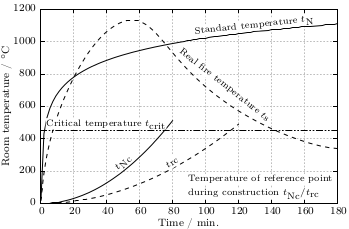Fig. 1 Fire severity as given by the fire temperature over time for a real vs. normalized fire. Click on the figure to see the original PDF version. (code to produce this figure, data)

To add a background to the labels we use the colorbox command, which we include in our terminal definition via the header option.

set terminal cairolatex standalone pdf size 16cm,10.5cm dashed transparent \


In addition, we specify the size of the background area with the \setlength{\fboxsep}{0.75pt} command. This is quite handy as the default background size of \colorbox is a little to large for labels.

For the labels themselves, we only have to highlight them with the \hl{} command to get the desired background.

set label 1 at  50, 250 '\hl{\small $t_\textrm{Nc}$}' center rotate by 45 front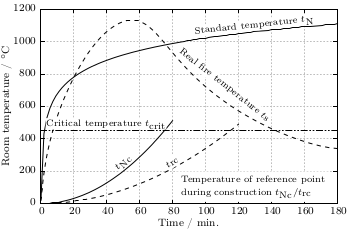Fig. 2 Fire severity as given by the fire temperature over time for a real vs. normalized fire. Click on the figure to see the original PDF version.(code to produce this figure, data)

If you have a label with a line break, you have to decide if you want to apply the background to every part of the line break, as shown in Fig. 1

set label 2 at  90, 100 '\small \shortstack[l]{\hl{Temperature of reference '.\
'point} \\ \hl{during construction $t_\textrm{Nc} / '.\ 't_\textrm{rc}$}}' front


or if you want to highlight the whole label without seeing some grid between the lines

set label 2 at  90, 100 '\hl{\small \shortstack[l]{Temperature of '.\
'reference point \\ during construction '.\
'$t_\textrm{Nc} / t_\textrm{rc}$}}' front


Fig. 2 shows the result for that one.

October 24th, 2013 | 4 Comments

If you want to put labels into a graph using the epslatex terminal you are probably interested in using a smaller font for these labes than for the rest of the figure. An example is presented in Fig. 1.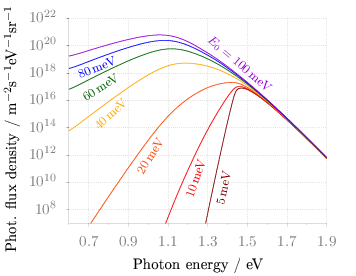Fig. 1 Photon flux density for different characteristic tail state energies E0 dependent on the photon energy. (code to produce this figure, data)

Figure 1 shows again the photon flux density from one of the last posts, but this time plotted with the epslatex terminal. The label size is changed by setting it to \footnotesize with the following code. First we introduce a abbreviation for the font size by adding a command definition to the header of our latex file.

set terminal epslatex size 9cm,7cm color colortext standalone header \
"\\newcommand{\\ft}{\\footnotesize}"


After the definition of the abbreviation we can use it for every label we are interested in.

set label 2 '\ft $5$\,meV'         at 1.38,4e9     rotate by  78.5 center tc ls 1
set label 3 '\ft $10$\,meV'        at 1.24,2e10    rotate by  71.8 center tc ls 2
set label 4 '\ft $20$\,meV'        at 1.01,9e11    rotate by  58.0 center tc ls 3
set label 5 '\ft $40$\,meV'        at 0.81,1e15    rotate by  43.0 center tc ls 4
set label 6 '\ft $60$\,meV'        at 0.76,9e16    rotate by  33.0 center tc ls 5
set label 7 '\ft $80$\,meV'        at 0.74,2.5e18  rotate by  22.0 center tc ls 6
set label 8 '\ft $E_0 = 100$\,meV' at 1.46,5e18    rotate by -40.5 center tc ls 7


September 8th, 2011 | 3 Comments

In the last entry about attractive plots we introduced the usage of gray borders and tics in order to emphasize the content of the figure.
If we want to do the same in the epslatex terminal, we had to add the color of the text directly with some LaTeX code:

set terminal epslatex size 10.4cm,6.35cm color colortext standalone \
set format '\color{tics}$%g$'


The problem is that the output looks not the way it should be, but like the one in Fig. 1.
It appears that all the letters we have written in the format string are used for the calculation of the size of the tics.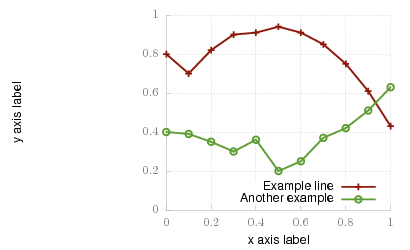Fig. 1 Colored tics plotted with Gnuplot version <4.4.3 (code to produce this figure)

An easy solution to that problem is to use Gnuplot 4.4.3, because the size calculation has been updated. Fig. 2 shows the result if we use Gnuplot 4.4.3 to create the figure. But still there is some room for improvements.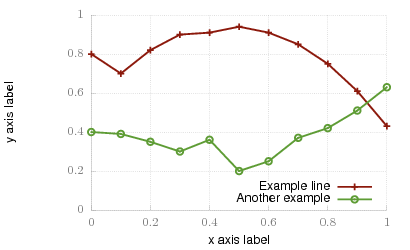Fig. 2 Colored tics plotted with Gnuplot 4.4.3 (code to produce this figure)

The length of the color name has still an influence on the calculated tics size. Hence we use only one letter for the color definition:

set terminal epslatex size 10.4cm,6.35cm color colortext standalone \
set format '\color{t}$%g$'


The final result is shown in Fig. 3.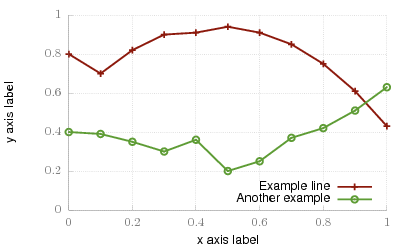Fig. 3 Colored tics plotted with Gnuplot 4.4.3 (code to produce this figure)

August 19th, 2011 | No Comments

I have updated the epslatex section in the introduction regarding output-terminals. Now it contains a hint how to deal with the problem of the right size of your plot and the font of the figure in a LaTeX document.

In addition I corrected some links to the Gnuplot documentation. Due to the fact that for the newer versions of Gnuplot no HTML-documentation is anymore available I had to link to the general documentation side instead of directly linking to the topic. It’s a pity and I will try to compile the documentation as HTML by myself and add it to this site.

May 20th, 2011 | 4 Comments

The tikz and epslatex terminal directly write text in the graph by using LaTeX. But if you want a line break within a label, a simple \\ will not work. In order to get a line break we can apply the \shortstack command.

set label '\shortstack{first line\\second line}'


In the following example, a principal component analysis (PCA) for attribute ratings of a listening test has been computed and the attributes together with the presented stimuli are plotted in the graph. The natural + little comb filter attribute and its counterpart included a line break (click on the figure for a larger version).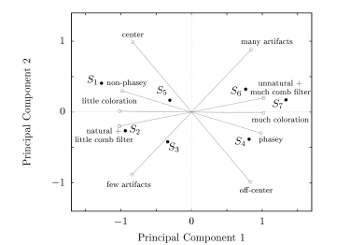Fig. 1 A PCA plot with a line break in two of its attribute labels (click on the figure for a larger view, code to produce this figure)

February 28th, 2011 | No Comments

There is a little update to the epslatex terminal introduction page.

The tics labels can automatically use the math mode from LaTeX by setting the right format option:

set format '$%g$'


Another change is to omit the \resizebox command in LaTeX to include the figure. The problem is that this command also scales your fonts, which you probably don’t want. The alternative is to specify the correct size of your plot before hand and then including the file only with:

\begin{figure}
\input{file}
\end{figure}


April 27th, 2010 | 53 Comments

Gnuplot gives us the opportunity to produce great looking plots in a lot of different formats. Therefore it uses different output terminals that can produce output files or as in the last chapter display the output on your computer screen. In this tutorial we will cover the png, svg, postscript and epslatex terminals. Therefore we will use the same code as in the previous plot functions chapter. We already used the wxt terminal there which displays the result on the screen:

set terminal wxt size 350,262 enhanced font 'Verdana,10' persist


## png, and svg terminal

The png and svg terminals produce more or less the same looking output as the wxt terminal, so you only have to replace the line for the wxt terminal with

set terminal pngcairo size 350,262 enhanced font 'Verdana,10'
set output 'introduction.png'


for png and with

set terminal svg size 350,262 fname 'Verdana' fsize 10
set output 'introduction.svg'


for svg to produce the same figures I have posted in the last chapter. You may have noticed that we use the pngcairo terminal. It exists also a png terminal, but it produces uglier output and doesn’t use the UTF-8 encoding the cairo library does. You may also have noticed that we set the size to a given x,y value. If we don’t do this, the default value of 640,480 is used. The enhanced option tells the terminals that they should interpret something like n_1 for us. But be aware that not all terminals handle the enhanced notation as mentioned in the gnuplot documentation.
Since we want to create an output file in both cases we have to specify one.
The png and svg terminals work very well to produce figures to use on the web as you can see on this page, but for scientific papers or other stuff written in LaTeX we would like to have figures in postscript or pdf format. I always create my pdf files of the plots from the postscript files, so I will cover only the postscript terminals in this introduction.

## postscript terminal

gnuplot has a postscript terminal that can be used to produce figures in the eps format:

set terminal postscript eps enhanced color font 'Helvetica,10'
set output 'introduction.eps'


But this creates very tiny fonts, as stated in the documentation:

In eps mode the whole plot, including the fonts, is reduced to half of the default size.

So if we want to produce the same font and line width dimensions, we have to use this command:

set terminal postscript eps size 3.5,2.62 enhanced color \
font 'Helvetica,20' linewidth 2


It tells the terminal the size in inches of the plot (note that this is the pixel dimension divided by 100) and uses a font size of 20 instead of 10. In addition to that it doubles the given line widths by a factor of 2.

Besides, the postscript terminal can’t handle all UTF-8 input characters and we have to use the enhanced mode to produce greek letter etc. But in opposite to the before mentioned terminals the enhanced mode will work fully for the postscript terminal.

set xlabel '{/Helvetica-Oblique x}'
set ylabel '{/Helvetica-Oblique y}'
set xtics ('-2{/Symbol p}' -2*pi,'-{/Symbol p}' -pi,0, \
'{/Symbol p}' pi,'2{/Symbol p}' 2*pi)
plot f(x) title 'sin({/Helvetica-Oblique x})' with lines linestyle 1, \


Finally, we will get the following figure. Note, that I have converted the resulting eps file to png with Gimp using strong text and graphics antialiasing to show it here. But the overall looking should be the same as with the original eps file.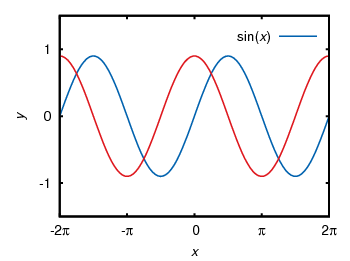Fig. 1 Sinusoid plotted using the postscript terminal (code to produce this figure)

If we compare Fig. 1 to the original png file made with the pngcairo terminal (Fig. 2) we see that we still have some changes. We need to adjust the tic scales and line widths by hand to get exactly the same result. Note: the font is of course different, because we used another one.Fig. 2 Sinusoid plotted using the pngcairo terminal (code to produce this figure)

This means we should consider if want to create svg and png images or if we want to create eps and png images. In the first case we will use the wxt, svg and pngcairo terminals together.
In the second case we will use only the postscript terminal and create the png files using Gimp or some other image processor.
You may think the handling of symbols e.g. greek letters is very weird in the postscript terminal and you are probably right. But you can also write symbols in LaTeX notation, therefore you have to use the epslatex terminal.

## epslatex terminal

If you have to write formulas or a scientific paper you are mostly interested in postscript/pdf or LaTeX output. The best way is to use both of them. That means to producing the lines etc. in the figure as a postscript image, but generate also a tex file that adds the text for us. The postscript image can be easily converted to a pdf using epstopdf if you needed a pdf version of the image. In order to create the tex file containing our figure labels we will use the epslatex terminal. Basically it has two working modes, a standalone mode that can produce a standalone postscript figure and the normal mode that produces a postscript figure and a tex file to include in your LaTeX document. I think the normal mode is the more common application so I will start with this.

For the normal mode we use the following terminal definition:

set terminal epslatex size 3.5,2.62 color colortext
set output 'introduction.tex'


color and colortext are needed if you have some colored labels, text etc. in your plot. size specifies the size of your plot in inches. You can specify the size alternatively in cm:

set terminal epslatex size 8.89cm,6.65cm color colortext


The easiest way is to specify the size to a value that corresponds with the needed one in your latex document. One way to get these values is to set your view to 100% in your pdf-viewer and measure the size of the area which should contain your figure. In Linux this is easily done using ScreenRuler.

Now we can write the labels etc. in LaTeX notation:

set xlabel '$x$'
set ylabel '$y$'
set format '$%g$'
set xtics ('$-2\pi$' -2*pi,'$-\pi$' -pi,0,'$\pi$' pi,'$2\pi$' 2*pi)
plot f(x) title '$\sin(x)$' with lines linestyle 1, \


Note that it is necessary to use the '' quotes and not "" in order to have interpreted your LaTeX code correctly.

If we run gnuplot on this file, it will generate a introduction.tex and a introduction-inc.eps file. In the LaTeX document we can then include the figure by:

\begin{figure}
\input{introduction}
\end{figure}


Now our figure uses automatically the font and font size of our paper, but we still have to check that the line widths and tics have the size we want to. Also the size of the figure itself has to be correct in order to include it with the \input command without resizing it. Therefore we had to know the size of our figure before hand as mentioned above. Otherwise you should use the \resizebox command in LaTeX, but then it could be that your font size will be incorrect.

\begin{figure}
\resizebox{\columnwidth}{!}{\input{introduction}}
\end{figure}


Note that you need the color package for colored labels. Therefore put \usepackage{color} in the preamble.

If you haven’t any LaTeX document, but want only to produce a figure with LaTeX labels, you can use the standalone mode.
Therefore exchange the above code with

set terminal epslatex size 3.5,2.62 standalone color colortext 10
set output 'introduction.tex'


The last value is the font size of the plot, which you don’t need if you want to include the figure in your LaTeX document.

To produce the standalone figure we have to run more than just the gnuplot command:

$gnuplot introduction.gnu$ latex introduction.tex
\$ dvips -o introduction.ps introduction.dvi


The first command results in two files: introduction.tex with a LaTeX header and introduction-inc.eps which is the eps file of the figure without any text. The latex command combine the two to a single dvi file and dvips generates a postscript file. Finally I have converted the postscript file with Gimp and we will get this png file:Fig. 3 Sinusoid plotted using the epslatex terminal (code to produce this figure)

If we compare this to Fig. 2 it is obvious that we have to fine tune the line widths and tics to get the same result as for the pngcairo terminal. In order to correct it we take the following values:

set border linewidth 2
set style line 1 linecolor rgb '#0060ad' linetype 1 linewidth 5
set style line 2 linecolor rgb '#dd181f' linetype 1 linewidth 5
set tics scale 1.25


Applying these settings will finally yield to a figure which looks like it should:Fig. 4 Sinusoid plotted using the epslatex terminal with corrected line width and tics (code to produce this figure)